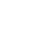Live chatThe family unit of U.S. Marine is outraged after a Michigan Adventure Park insisted on charging every quadriplegic person entering the park, despite being a quadriplegic marine. The Michigan Adventure Manager said that the park rules require that every person admitted should pay for the services provided. He further argues that the number of quadriplegic marine has been rising rapidly for the past few years. They increase the cost of the organization and compel the company to employ more caregivers. Therefore, the company manager wants to determine the number of quadriplegic marine admitted per week. He randomly selected the data of twelve weeks and write down the following. 20, 22, 22, 21, 18, 12, 30, 26, 24, 17, 22, 20. The data above can be represented in the form of a histogram as shown below.

The height of the histogram (y-axis) reflects the number of quadriplegic marine admitted per week. The x-axis shows the number of weeks. The data statistics are extending out across the horizontal axis of the diagram.Central limit theorem tends to explain why many distributions are close to the normal distribution. Plotting data in a histogram distribution shows the common figure of the distribution of the quadriplegic marine admitted to Michigan adventure. Its gives a general sense of the numbers are bunched. Manager can opt to use several methods of statistic to determine the center of distribution. In addition, he will determine the degree to which individuals admitted diverge from the standard value in a distribution.

The data figures are spread out across the horizontal axis of the graph, but median, mean and mode are all clustered toward the center. Median is the number of which lies at the centre when a series of data is arranged in ascending and descending order. According to the data provided above, 21 represent the median number of quadriplegic marine admitted per week. It is calculated by arranging the number in ascending order as follows. 12, 17, 18, 20, 20, 21, 22, 22, 22,24,26,30, and then take median number. The median number is 21.5, but since the manager is calculating the number of people round off to 22.

Limited time Offer

0
0
days
:
0
0
hours
:
0
0
minutes
:
0
0
seconds
Get 19% OFF

The sample mean is also referred to as the average number. The shape of histogram above is approximately identical on the right face and the left side of the diagram. Hence, this data is symmetrical. Mean is the best measure of the central tendency. It is calculated by adding the data collected and then divide by the sum amount of outcomes(Oliver Johnson, 2004). Mean is the descriptive statistics mostly used in calculating the measure of central tendency. From the data above, manager calculate the mean as follows. 12+17+18+20+20+21+22+22+22+24+26+30=254. Then divide the total value by 12 to get 21. 5. Therefore, the manager can conclude that the average number of quadriplegic marine admitted per week is 21.

Mode is that value of the statistics which occurs most regularly. If the data has one modal value it is referred to as unimodal value, and if it has two values it is termed to as bimodal data. From the histogram above, 22 represent the most frequent number. Therefore, the most frequent number of quadriplegic marine admitted per weeks is 22. Hence, the manager can conclude that from the measures of central tendency 22 and 21 are the most frequent number of quadriplegic marine admitted per week.

Stay ConnectedVariance is a determinant of arithmetical dispersion representing how values are spread around the expected value or arithmetic mean. It is calculated as follows.

## Number of 12 17 18 20 20 21 22 22 22 24 26 30

(x-21.6) -9.2 -4.2 -3.2 -1.2 -1.2 -02 0.8 0.8 0.8 2.8 4.8 8.8

(x-21.2) ^2 84.6 17.6 10.2 1.4 1.2 0.04 0.6 0.6 0.6 7.8 23 77

Hence, 225.3/12=18.8

Standard deviation is the cube roots of the arithmetic mean of the squared divergence from the arithmetic mean. It is computed the same way as variance, but we get the square root of the variance the mean. 4.3. Since the standard deviation measure how the data value deviates from the mean, then the manager will go one standard deviation in any direction. The mean is 21.5, and standard deviation is 4.3. 21-4.3=17.2 and 21.5+4.3=25.5. This mean that most of the quadriplegic marine between 17 and 25.

0

Preparing Orders

0

Active Writers

0%

Positive Feedback

0

Support Agents

Now Accepting Apple Pay!
Limited offerget 15% off your 1st order with code first15